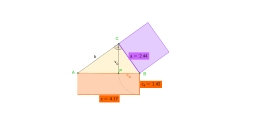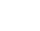# Free space in the garden

The grandfather's free space in the garden was in the shape of a rectangular triangle with 5 meters and 12 meters in length. He decided to divide it into two parts and the height of the hypotenuse. For the smaller part creates a rock garden, for the larger sows grass. How many square meters has a larger part?

Correct result:

S =  25.5621 m2

#### Solution:We would be pleased if you find an error in the word problem, spelling mistakes, or inaccuracies and send it to us. Thank you!Tips to related online calculators
Pythagorean theorem is the base for the right triangle calculator.

#### You need to know the following knowledge to solve this word math problem:

We encourage you to watch this tutorial video on this math problem:

## Next similar math problems:

• Rectangular trapezoidThe ABCD rectangular trapezoid with the AB and CD bases is divided by the diagonal AC into two equilateral rectangular triangles. The length of the diagonal AC is 62cm. Calculate trapezium area in cm square and calculate how many differs perimeters of the
• Top of the towerThe top of the tower has the shape of a regular hexagonal pyramid. The base edge has a length of 1.2 m, the pyramid height is 1.6 m. How many square meters of sheet metal is needed to cover the top of the tower if 15% extra sheet metal is needed for joint
• Horses playgroundThe fence for the horses has the shape of a rectangular trapezoid with an area of 400 m2, the base lengths should be 31 m and 19 m. How many meters of boards will they need to fence it if the boards are stacked in 5 rows?
• Roof coverAbove the pavilion with a square ground plan with a side length of a = 12 m is a pyramid-shaped roof with a height v = 4.5 m. Calculate how much m2 of sheet metal is needed to cover this roof if 5.5% of the sheet we must add for joints and waste.
• Base of prismThe base of the perpendicular prism is a rectangular triangle whose legs length are at a 3: 4 ratio. The height of the prism is 2cm smaller than the larger base leg. Determine the volume of the prism if its surface is 468 cm2.
• A cylinderA cylinder 108 cm high has a circumference of 24 cm. A string makes exactly 6 complete turns around the cylinder while its two ends touch the cylinder's top and bottom. (forming a spiral around the cylinder). How long is the string in cm?
• Rectangular gardenThe sides of the rectangular garden are in ratio 1: 2. The diagonal has a length of 20 meters. Calculate the area and perimeter of the garden.
• The tractorThe tractor sows an average of 1.5 ha per hour. In how many hours does it sows a rectangular trapezoid field with the bases of 635m and 554m and a longer arm 207m?
• Triangular prismThe base of the perpendicular triangular prism is a rectangular triangle with a hypotenuse of 10 cm and one leg of 8 cm. The prism height is 75% of the perimeter of the base. Calculate the volume and surface of the prism.
• Digging a pitThe pit has the shape of a regular quadrilateral truncated pyramid. The edges of the bases are 14m and 10m long. The sidewalls form an angle of 135° with a smaller base. Determine how many m3 of soil were excavated when digging the pit?
• Right triangular prismWe have cuboid with a base and dimensions of 12 cm and 5 cm and height of 4 cm. The tablecloth cut it into two identical triangular prisms with right triangular bases. The surface of the created prisms was painted with color. Calculate the surface area of
• The gardenThe garden has the shape of a rectangular trapezium. The bases have lengths of 27 meters and 36 meters, the trapezoid's height is 12 meters. Calculate how much fence will cost this garden if one meter costs 1.5 €?
• Squares above sidesTwo squares are constructed on two sides of the ABC triangle. The square area above the BC side is 25 cm2. The height vc to the side AB is 3 cm long. The heel P of height vc divides the AB side in a 2: 1 ratio. The AC side is longer than the BC side. Calc
• Isosceles trapezoidThe old father decided to change the top plate of an isosceles-like trapezoid with the basic dimensions of 120 cm and 60 cm, and the shoulder is 50 centimeters long. How much does it pay for a new plate and a square meter worth 17 euros?
• Right ΔA right triangle has the length of one leg 11 cm and length of the hypotenuse 61 cm. Calculate the height of the triangle.
• R TrapeziumRectangular trapezium has bases 12 and 5 and area 84 cm2. What is its perimeter?
• RhumblineFind circumference and area of the rhumbline ABCD if the short side AD of which has a length of 5 cm, and the heel of the height from D leading to the AB side divides the AB side into two sections of 3 cm and 4 cm.